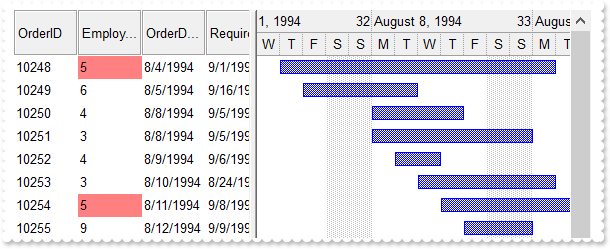property ConditionalFormat.ApplyTo as FormatApplyToEnum
Specifies whether the format is applied to items or columns.Type Description FormatApplyToEnum A FormatApplyToEnum expression that indicates whether the format is applied to items or to columns. If the ApplyTo property is less than zero, the format is applied to the items.
By default, the format is applied to items. The ApplyTo property specifies whether the format is applied to the items or to the columns. If the ApplyTo property is greater or equal than zero the format is applied to the column with the index ApplyTo. For instance, if the ApplyTo property is 0, the format is applied to the cells in the first column.  If the ApplyTo property is 1, the format is applied to the cells in the second column, if the ApplyTo property is 2, the format is applied to the cells in the third column, and so on. If the ApplyTo property is -1, the format is applied to items.  The ApplyToBars property specifies the list of bars that the current format is applied to.

The following screen shot shows a conditional expression applied to items:The following screen shot shows a conditional expression applied to columns:The following VB sample bolds the cells in the second column ( 1 ), if the sum between second and third column ( 2 ) is less than the value in the first column ( 0 ):

```With G2antt1.ConditionalFormats.Add("%1+%2<%0")
.ApplyTo = 1
.Bold = True
End With```

The following C++ sample bolds the cells in the second column ( 1 ), if the sum between second and third column ( 2 ) is less than the value in the first column ( 0 ):

```COleVariant vtEmpty;
CConditionalFormat cf = m_g2antt.GetConditionalFormats().Add( "%1+%2<%0", vtEmpty );
cf.SetBold( TRUE );
cf.SetApplyTo( 1 );```

The following VB.NET sample bolds the cells in the second column ( 1 ), if the sum between second and third column ( 2 ) is less than the value in the first column ( 0 ):

```With AxG2antt1.ConditionalFormats.Add("%1+%2<%0")
.ApplyTo = 1
.Bold = True
End With```

The following C# sample bolds the cells in the second column ( 1 ), if the sum between second and third column ( 2 ) is less than the value in the first column ( 0 ):

```EXG2ANTTLib.ConditionalFormat cf = axG2antt1.ConditionalFormats.Add("%1+%2<%0",null);
cf.Bold = true;
cf.ApplyTo = (EXG2ANTTLib.FormatApplyToEnum)1;```

The following VFP sample bolds the cells in the second column ( 1 ), if the sum between second and third column ( 2 ) is less than the value in the first column ( 0 ):

```with thisform.G2antt1.ConditionalFormats.Add("%1+%2<%0")
.Bold = .t.
.ApplyTo = 1
endwith```